## 3.21Terms which change sign

An equation may include a function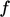which can return a value which is positive or negative, e.g.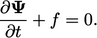(3.37)
Following Sec. 3.20 , we aim to avoid a singular matrix in the case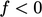and ensure the solution exceeds the lower bound of 0 when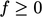. The discretisation of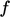is therefore treated implicitly or explicitly within each cell based on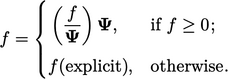(3.38)

### Maintaining lower and upper bounds

The solution variable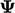could have a physical bound at any low value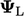and/or high value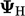. We can treat the functionin Eq. (3.37 ) as follows to maintain boundedness: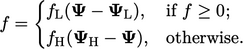(3.39)
Here,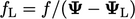and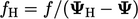are calculated using the current values of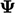.

If we examine the situation with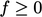, Eq. (3.37 ) would be discretised as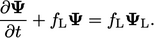(3.40)
When we discretise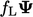implicitly alongside the Euler time scheme, Eq. (3.40 ) becomes: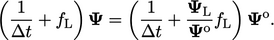(3.41)
The solution fordecreases when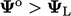, but cannot fall below the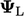bound within a solution step. In the limit that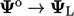, the solution forno longer decreases.

An equivalent analysis shows Eq. (3.40 ) maintains boundedness for the upper bound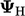. In that case, note that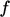is negative so the term in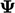can still be treated implicitly.

### Fields bounded by 0 and 1

Many properties are expressed as a fraction, e.g. concentration of a chemical species, so are bounded by 0 and 1. When that happens, Eq. (3.39 ) simpliﬁes to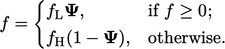(3.42)
Here,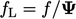and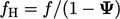are calculated using the current values of.

It is particularly important to maintain boundedness of a ﬁeld with 0-1 bounds when it is used to calculate another property which has a physical bound. For example, in a system ofﬂuid phases, ﬂuid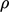can be calculated by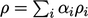from phase fractions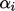and densities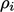.

A small amount of unboundedness in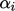, in the phase with highest, causes large unboundedness in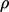, e.g. in 2 phases with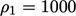and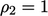, the calculated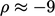with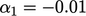.

Notes on CFD: General Principles - 3.21 Terms which change sign